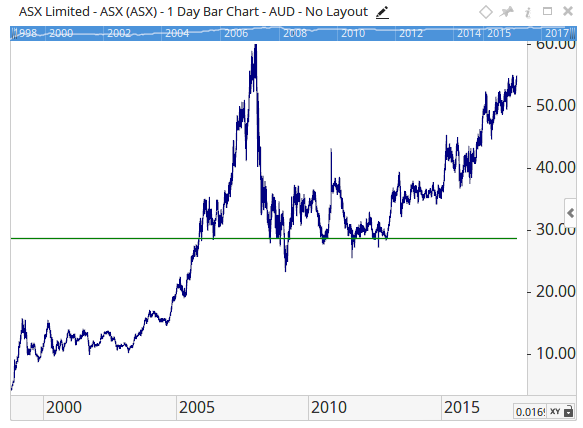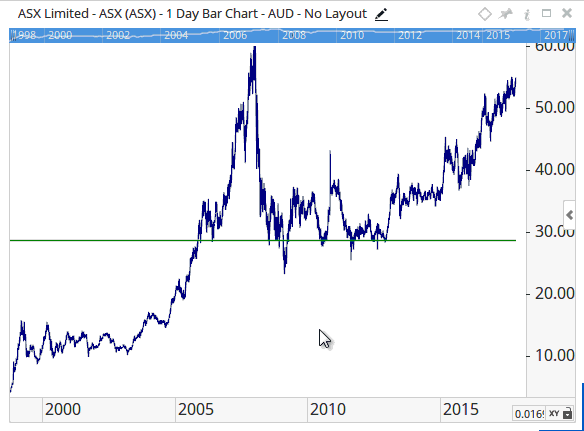# BarCount Function

Author: Optuma Team Last updated: Oct 26, 2018 02:05

## Overview

The `BarCount()` script function will return either the total number of data bars that are available for a symbol, or the total number of bars on the screen at the time. This can be used in instances where you want to disregard newly floated stocks, or wanting to have a dynamic moving average calculated off the total number of bars in view.

For example, if you wanted a scan to disregard newly listed stocks you could use something like this:

``````BarCount() > 100
``````

This would mean that if a chart had less than 100 trading days it would be excluded from the scans results.

This example can be used in a Show Plot to calculate an average price level based off the entire chart’s price history.

``````\$b = BARCOUNT();
v1 = MA(BARS=\$b);
LAST(V1)
``````

On the chart the result would look like this, with the horizontal line being the average of the entire history of the chart:This script can be modified so that the average price is calculated based off the number of bars visible in the screen at the time:

``````\$b = BARCOUNT(RANGE=VISIBLE);
v1 = MA(BARS=\$b);
LAST(V1)
``````As you zoom in / out and the number of bars in view changes, the line will be recalculated.